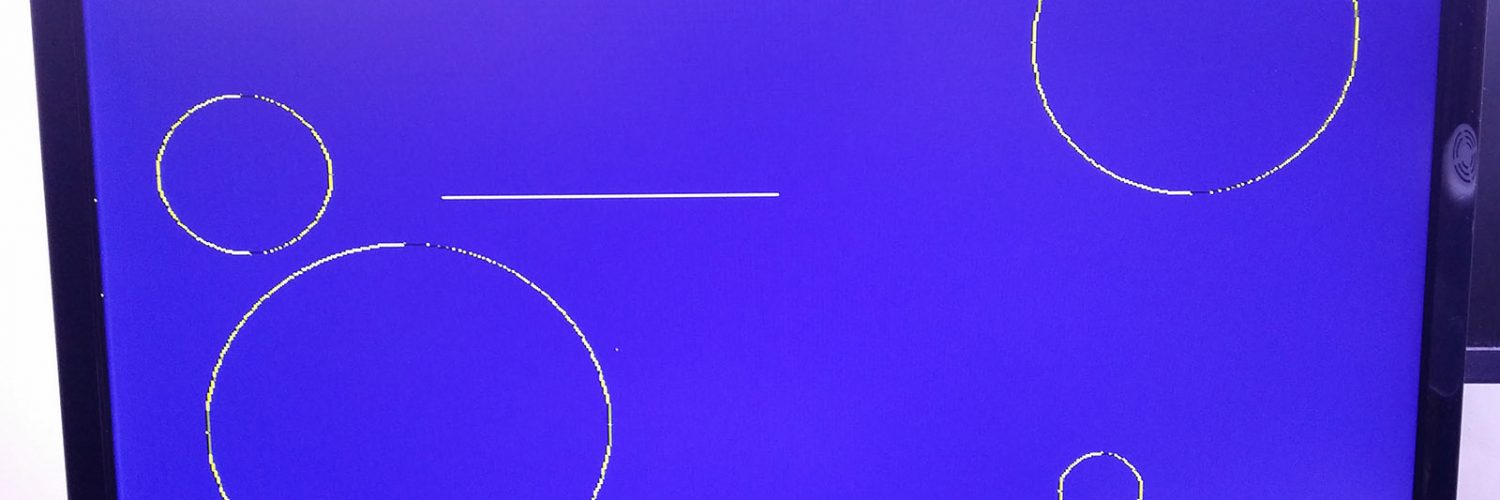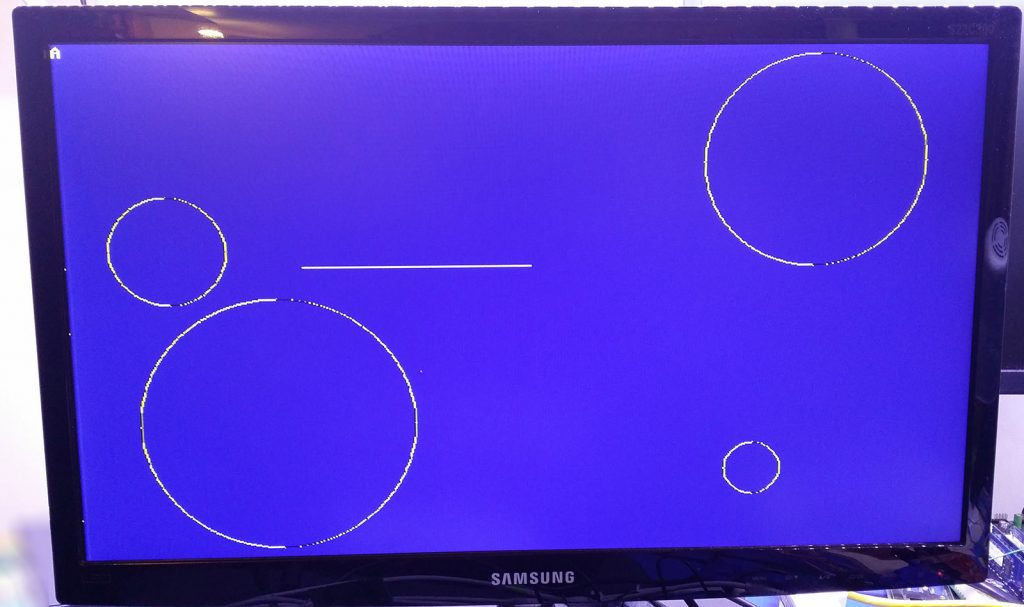# Graphics Primitives are working, at least mostly.So we’ve got a frame buffer now which means we can start writing 68K routines to write graphics into it.

So here I’m playing around with lines and circles. I’m using a random line drawing routine from the Atari that I found. I’m using the C code from the Midpoint Circle Algorithm on wikipedia, that has been compiled, and then has the assembly modified to work without support for negative numbers.

I’m seeing some graphic defect, but I’m not sure if that’s due to

• A defect in the assembly of the Midpoint Circle algorithm
• An existing cpu write problem (sharing SRAM)
• a video display issue (also sharing SRAM)
• an artifact of the built-in monitor upscaling algorithm

The C code runs fine in windows without any similar effect.

Here’s the code:

; }
; void drawcircle(short x0,short y0,short radius)
; {
_drawcircle:
lea _putpixel.L,A2
; short y = 0;
clr.w D2
; short err = 0;
;clr.w D6
move.l #\$8000, D6 ; this is an arbitrary number since we can't easily handle negative numbers.
; while (x &gt;= y)
drawcircle_1:
cmp.w D2,D3
blt drawcircle_3
; {
; putpixel(x0 + x, y0 + y);
move.w D5,D0
ext.l D0
move.w D4,D1
ext.l D1
jsr (A2)

; putpixel(x0 + y, y0 + x);
move.w D5,D0
ext.l D0
move.w D4,D1
ext.l D1
jsr (A2)

; putpixel(x0 - y, y0 + x);
move.w D5,D0
ext.l D0
move.w D4,D1
sub.w D2,D1
ext.l D1
jsr (A2)

; putpixel(x0 - x, y0 + y);
move.w D5,D0
ext.l D0
move.w D4,D1
sub.w D3,D1
ext.l D1
jsr (A2)

; putpixel(x0 - x, y0 - y);
move.w D5,D0
sub.w D2,D0
ext.l D0
move.w D4,D1
sub.w D3,D1
ext.l D1
jsr (A2)

; putpixel(x0 - y, y0 - x);
move.w D5,D0
sub.w D3,D0
ext.l D0

move.w D4,D1
sub.w D2,D1
ext.l D1
jsr (A2)

; putpixel(x0 + y, y0 - x);
move.w D5,D0
sub.w D3,D0
ext.l D0
move.w D4,D1
ext.l D1
jsr (A2)

; putpixel(x0 + x, y0 - y);
move.w D5,D0
sub.w D2,D0
ext.l D0
move.w D4,D1
ext.l D1
jsr (A2)

; if (err &lt;= 0) cmp.l #\$8000,D6 bgt.s drawcircle_4 ; { ; y += 1; addq.w #1,D2 ; err += 2*y + 1; move.w D2,D0 asl.l #1,D0 addq.w #1,D0 add.w D0,D6 drawcircle_4: ; } ; if (err &gt; 0)
cmp.l #\$8000,D6
ble.s drawcircle_6
; {
; x -= 1;
subq.w #1,D3
; err -= 2*x + 1;
move.w D3,D0
asl.l #1,D0
sub.w D0,D6
drawcircle_6:
bra drawcircle_1
drawcircle_3:
rts

You also see a remnant from my previous text generation in the upper left hand corner.

I’m open to thoughts on the circle problem!#### keith

Amateur Electronics Design Engineer and Hacker